# 从PHP客户端看MongoDB通信协议

### getNext与网络请求

```\$m = new Mongo();
\$collection = \$m->demoDb->demoCollection;
\$cursor = \$collection->find();```

```\$cursor->sort( array( 'name' => 1 ) );
\$result = \$cursor->getNext();```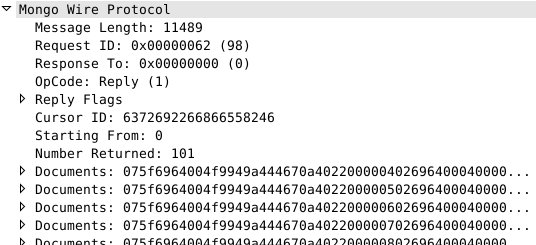```// skip the other 100 docs
for (\$i = 0; \$i < 100; \$i++) { \$cursor->getNext(); }
// request document 102:
\$result = \$cursor->getNext();```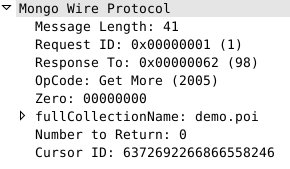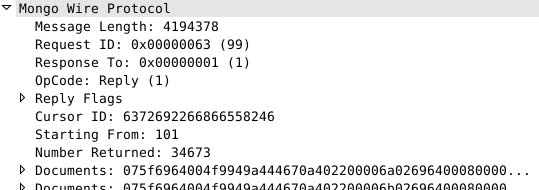### 设置batchSize

```\$cursor = \$collection->find()->sort( array( 'name' => 1 ) );
\$cursor->batchSize(25);
\$result = \$cursor->getNext();```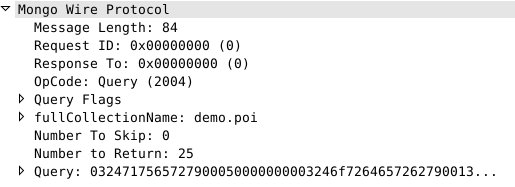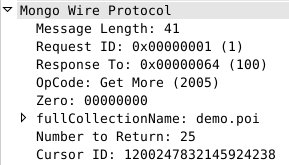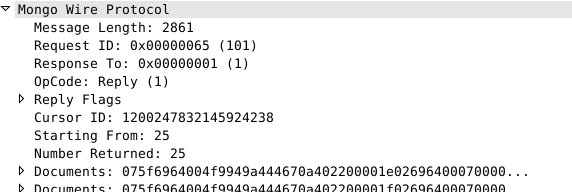### 使用limit

```\$cursor = \$c->find()->sort( array( 'name' => 1 ) );
\$cursor->limit( 50000 );
\$res = \$cursor->getNext();```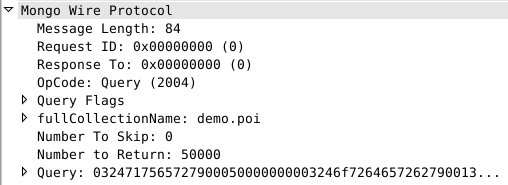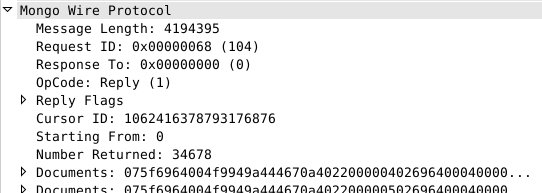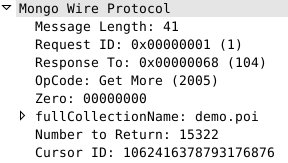### batchSize 和 limit 相组合

```\$cursor = \$c->find()->sort( array( 'name' => 1 ) );
\$cursor->limit( 128 )->batchSize( 50 );
\$res = \$cursor->getNext();
// retrieve the other 127 documents that we still want
for (\$i = 0; \$i < 127; \$i++) { \$cursor->getNext(); }```

### 关于 batchSize 函数的小问题

```\$cursor = \$c->find()->sort( array( 'name' => 1 ) );
\$cursor->batchSize( 1 )->limit( 10 );
\$cursor->getNext();
var_dump( \$cursor->getNext() );```

```\$cursor = \$c->find()->sort( array( 'name' => 1 ) );
\$cursor->batchSize( 2 )->limit( 10 );
\$cursor->getNext(); // item 1
\$cursor->getNext(); // item 2
var_dump( \$cursor->getNext() ); // item 3```